# MODULE 10 REVIEW Question 1 REVIEW REVIEW REVIEW

• Slides: 16MODULE 10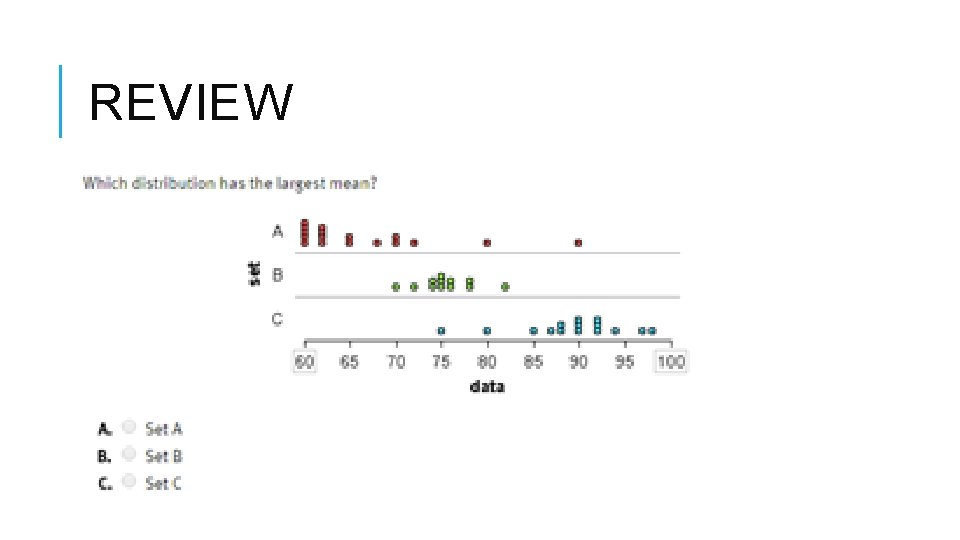REVIEW Question 1REVIEWREVIEW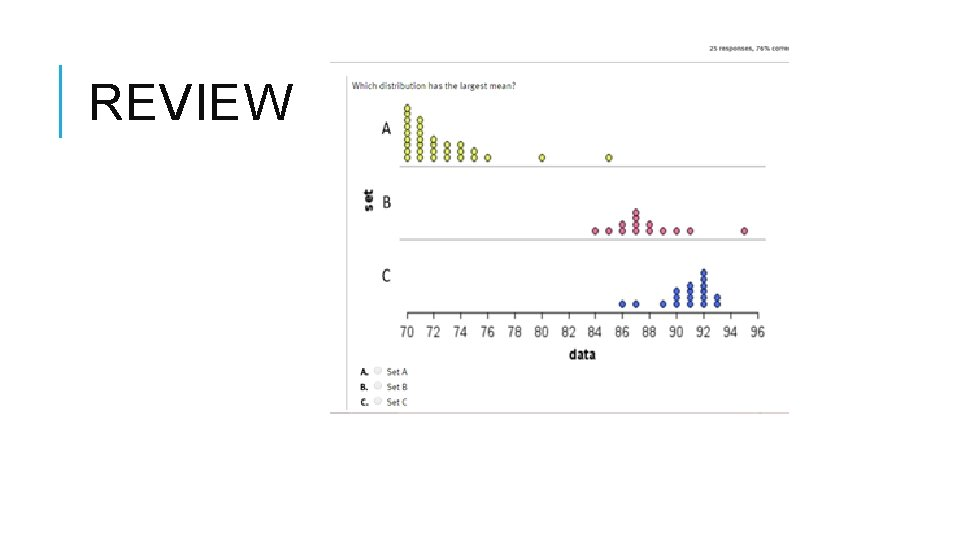REVIEW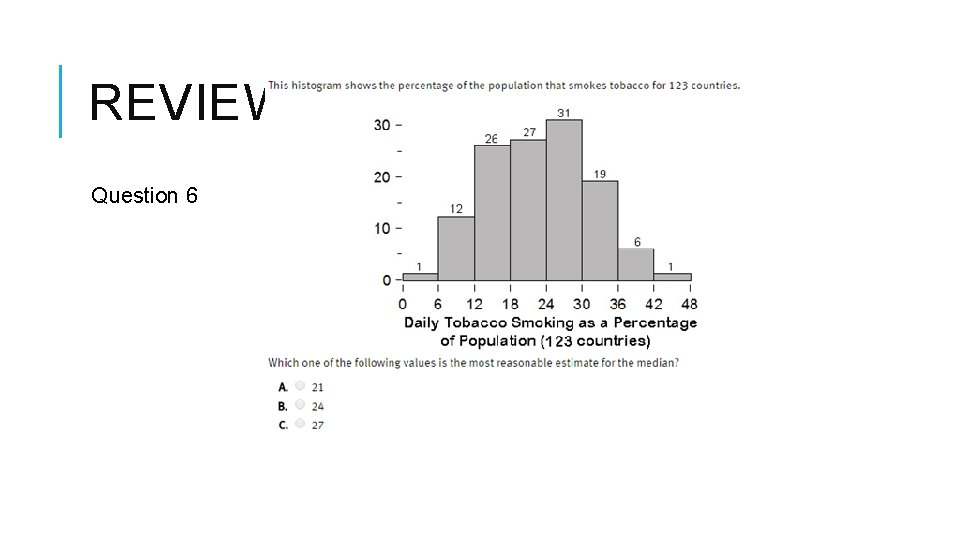REVIEW Question 6FIRST WE REVIEW What do you remember about box plots?LET’S DEBATE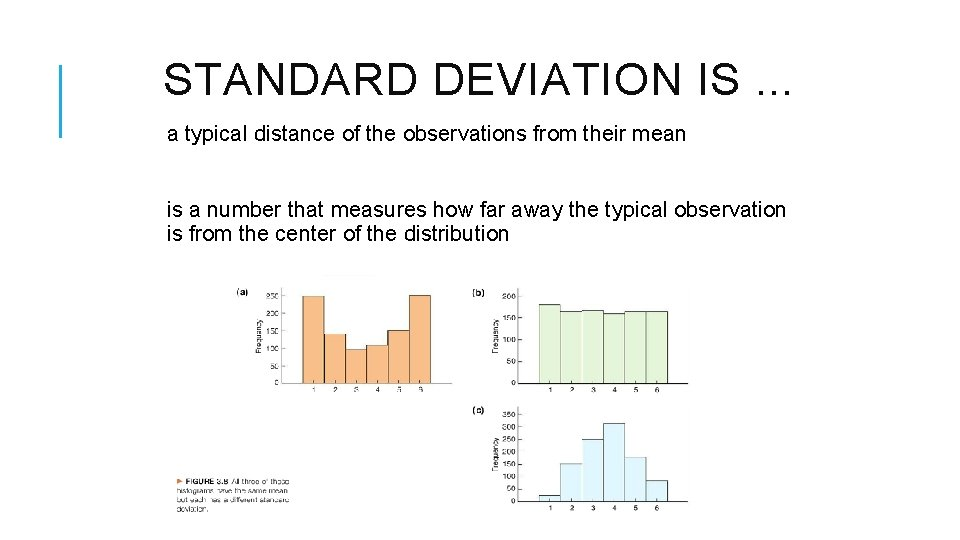STANDARD DEVIATION IS. . . a typical distance of the observations from their mean is a number that measures how far away the typical observation is from the center of the distribution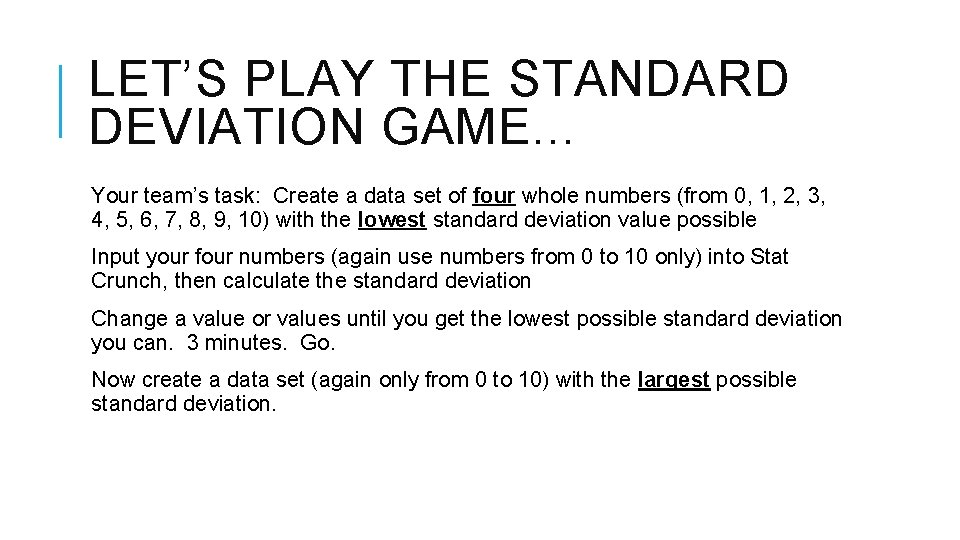LET’S PLAY THE STANDARD DEVIATION GAME. . . Your team’s task: Create a data set of four whole numbers (from 0, 1, 2, 3, 4, 5, 6, 7, 8, 9, 10) with the lowest standard deviation value possible Input your four numbers (again use numbers from 0 to 10 only) into Stat Crunch, then calculate the standard deviation Change a value or values until you get the lowest possible standard deviation you can. 3 minutes. Go. Now create a data set (again only from 0 to 10) with the largest possible standard deviation.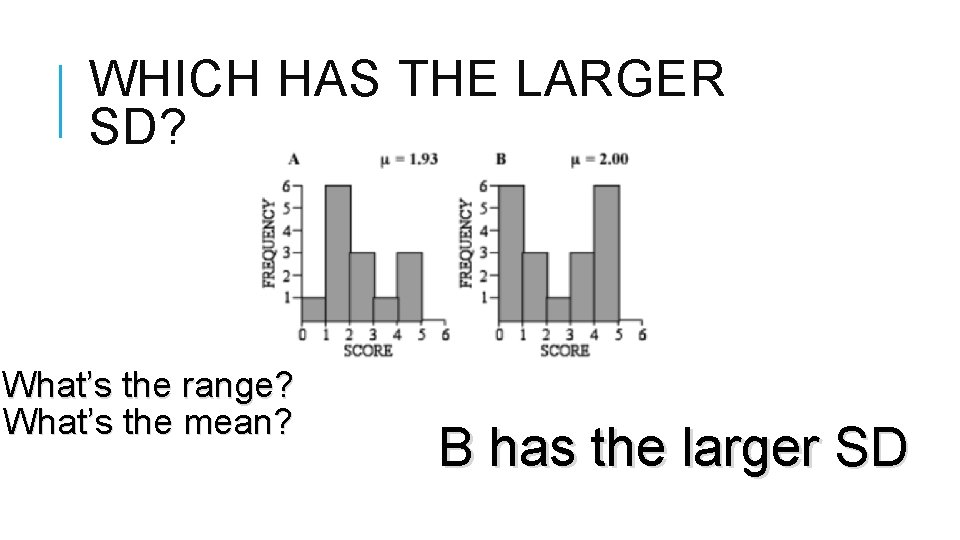WHICH HAS THE LARGER SD? What’s the range? What’s the mean? B has the larger SD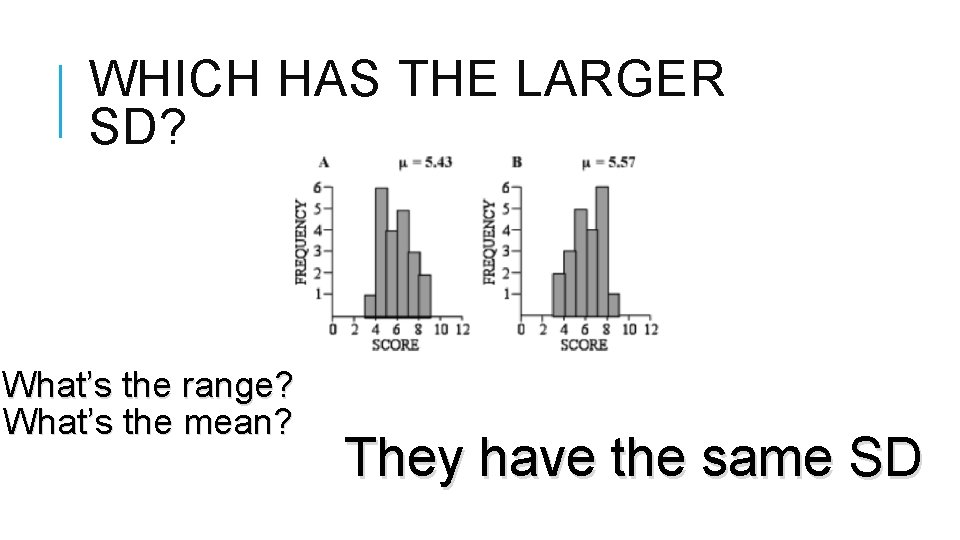WHICH HAS THE LARGER SD? What’s the range? What’s the mean? They have the same SD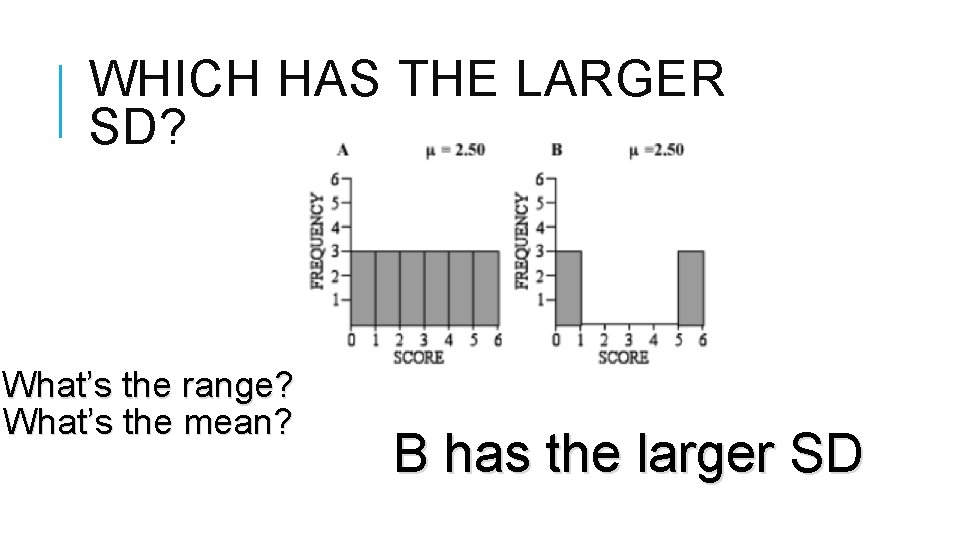WHICH HAS THE LARGER SD? What’s the range? What’s the mean? B has the larger SDWHICH HAS THE LARGER SD? What’s the range? What’s the mean? B has the larger SD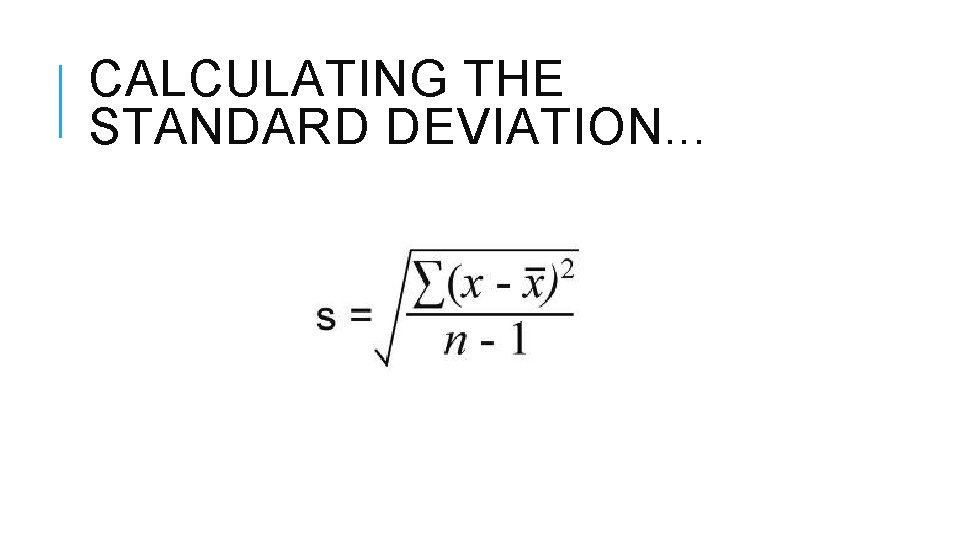CALCULATING THE STANDARD DEVIATION. . .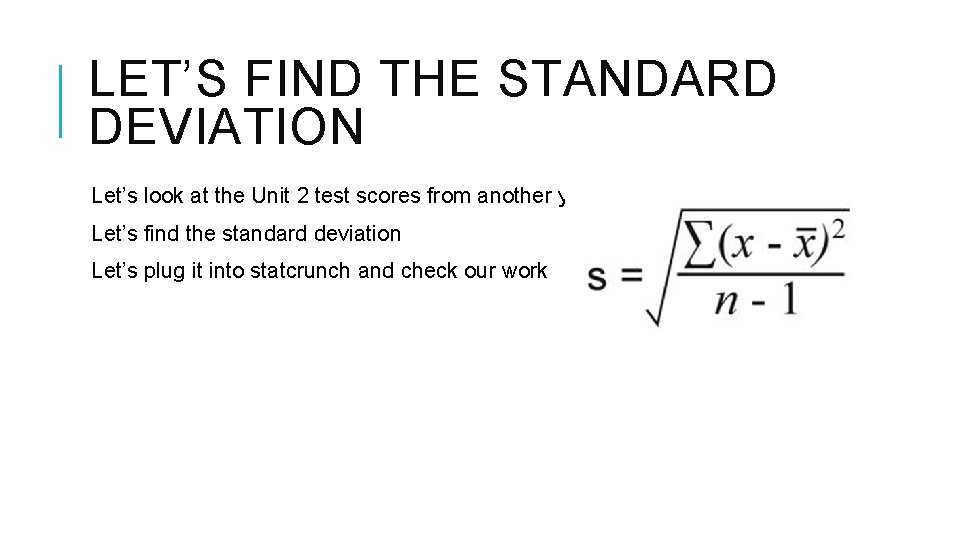LET’S FIND THE STANDARD DEVIATION Let’s look at the Unit 2 test scores from another year Let’s find the standard deviation Let’s plug it into statcrunch and check our work